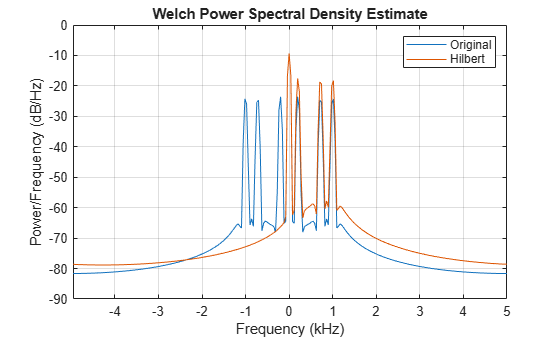Documentation

This is machine translation

Mouseover text to see original. Click the button below to return to the English version of the page.

Analytic Signal and Hilbert Transform

The `hilbert` function finds the exact analytic signal for a finite block of data. You can also generate the analytic signal by using an finite impulse response (FIR) Hilbert transformer filter to compute an approximation to the imaginary part.

Generate a sequence composed of three sinusoids with frequencies 203, 721, and 1001 Hz. The sequence is sampled at 10 kHz for about 1 second. Use the `hilbert` function to compute the analytic signal. Plot it between 0.01 seconds and 0.03 seconds.

```fs = 1e4; t = 0:1/fs:1; x = 2.5 + cos(2*pi*203*t) + sin(2*pi*721*t) + cos(2*pi*1001*t); y = hilbert(x); plot(t,real(y),t,imag(y)) xlim([0.01 0.03]) legend('real','imaginary') title('hilbert Function')```Compute Welch estimates of the power spectral densities of the original sequence and the analytic signal. Divide the sequences into Hamming-windowed, nonoverlapping sections of length 256. Verify that the analytic signal has no power at negative frequencies.

```pwelch([x;y].',256,0,[],fs,'centered') legend('Original','hilbert')```Use the `designfilt` function to design a 60th-order Hilbert transformer FIR filter. Specify a transition width of 400 Hz. Visualize the frequency response of the filter.

```fo = 60; d = designfilt('hilbertfir','FilterOrder',fo, ... 'TransitionWidth',400,'SampleRate',fs); freqz(d,1024,fs)```Filter the sinusoidal sequence to approximate the imaginary part of the analytic signal.

`hb = filter(d,x);`

The group delay of the filter, `grd`, is equal to one-half the filter order. Compensate for this delay. Remove the first `grd` samples of the imaginary part and the last `grd` samples of the real part and the time vector. Plot the result between 0.01 seconds and 0.03 seconds.

```grd = fo/2; y2 = x(1:end-grd) + 1j*hb(grd+1:end); t2 = t(1:end-grd); plot(t2,real(y2),t2,imag(y2)) xlim([0.01 0.03]) legend('real','imaginary') title('FIR Filter')```Estimate the power spectral density (PSD) of the approximate analytic signal and compare it to the `hilbert` result.

```pwelch([y;[y2 zeros(1,grd)]].',256,0,[],fs,'centered') legend('hilbert','FIR Filter')```